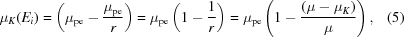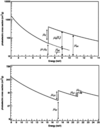JOURNAL OFSYNCHROTRONRADIATION
ISSN: 1600-5775
Volume 25| Part 2| March 2018| Pages 627-628

## Absorbed dose calculations for macromolecular crystals: improvements to RADDOSE. Erratum

aLaboratory of Molecular Biophysics, Department of Biochemistry, University of Oxford, South Parks Road, Oxford OX1 3QU, UK, and bSwiss Light Source, Paul Scherrer Institut, CH-5232 Villigen PSI, Switzerland
*Correspondence e-mail: elspeth.garman@bioch.ox.ac.uk

(Received 5 February 2018; accepted 5 February 2018)

Corrections to an equation and a figure in the paper by Paithankar et al. (2009). [J. Synchrotron Rad. 16, 152–162] are made.

Correct versions of equation (5) and Fig. 4 and its legend in the paper by Paithankar et al. (2009). [J. Synchrotron Rad. 16, 152–162] are given. The last line on page 155 and equation (5) on page 156 should read as follows:

The fraction of μpe attributable to K-shell ionization (above the K-edge), μK(Ei), at an incident X-ray energy Ei is equal to (see Fig. 4):where r is the `edge ratio', defined as μ/(μμK), and μ and μK are the total and K-shell photoelectric cross sections, respectively, at the K-edge.

(The revised version of Fig. 4is given overleaf.)Figure 4 The photoelectric cross section of iron (top) and uranium (bottom) as a function of energy. μK, μL and μM are the contributions of the K-, L- and M-shell cross sections to the total photoelectric cross section, and r is the edge ratio: r = μ/(μ − μK) and μ/(μ − μL) at any K- and L-edge, respectively.

### Footnotes

Present address: Diamond Light Source Ltd, Diamond House, Harwell Science and Innovation Campus, Didcot, Oxfordshire OX11 0DE, UK.

### Acknowledgements

We thank Joshua L. Dickerson (University of Oxford, UK) for pointing out the error in the original paper.

### ReferencesJOURNAL OFSYNCHROTRONRADIATION